Students can download 12th Business Maths Chapter 1 Applications of Matrices and Determinants Miscellaneous Problems Questions and Answers, Samacheer Kalvi 12th Business Maths Book Solutions Guide Pdf helps you to revise the complete Tamilnadu State Board New Syllabus and score more marks in your examinations.

## Tamilnadu Samacheer Kalvi 12th Business Maths Solutions Chapter 1 Applications of Matrices and Determinants Miscellaneous Problems

Question 1.
Find the rank of the matrix A = $$\left(\begin{array}{rrrr} 1 & -3 & 4 & 7 \\ 9 & 1 & 2 & 0 \end{array}\right)$$
Solution:
A = $$\left(\begin{array}{rrrr} 1 & -3 & 4 & 7 \\ 9 & 1 & 2 & 0 \end{array}\right)$$
Order of A is 2 × 4. So ρ(A) ≤ 2
Consider the second order minor
$$\left|\begin{array}{cc} 1 & -3 \\ 9 & 1 \end{array}\right|$$ = 1 + 27 = 28 ≠ 0
There is a minor of order 2 which is not zero.
So ρ(A) = 2

Question 2.
Find the rank of the matrix A = $$\left(\begin{array}{rrrr} -2 & 1 & 3 & 4 \\ 0 & 1 & 1 & 2 \\ 1 & 3 & 4 & 7 \end{array}\right)$$
Solution:
Given A = $$\left(\begin{array}{rrrr} -2 & 1 & 3 & 4 \\ 0 & 1 & 1 & 2 \\ 1 & 3 & 4 & 7 \end{array}\right)$$
Order of A is 3 × 4. So ρ(A) ≤ 3
Consider the third order minor
$$\left|\begin{array}{ccc} -2 & 1 & 3 \\ 0 & 1 & 1 \\ 1 & 3 & 4 \end{array}\right|$$
= -2(4 – 3) – 1(0 – 1) + 3(0 – 1)
= -2 + 1 – 3 =
= -4 ≠ 0
There exists a minor of order 3 which is not zero. So ρ(A) = 3

Question 3.
Find the rank of the matrix A = $$\left(\begin{array}{llll} 4 & 5 & 2 & 2 \\ 3 & 2 & 1 & 6 \\ 4 & 4 & 8 & 0 \end{array}\right)$$
Solution:
Given A = $$\left(\begin{array}{llll} 4 & 5 & 2 & 2 \\ 3 & 2 & 1 & 6 \\ 4 & 4 & 8 & 0 \end{array}\right)$$
Order of A is 3 × 4. So ρ(A) ≤ 3
Consider the third order minor,
$$\left|\begin{array}{lll} 4 & 5 & 2 \\ 3 & 2 & 1 \\ 4 & 4 & 8 \end{array}\right|$$
= 4(16 – 4) – 5(24 – 4) + 2 (12 – 8)
= 48 – 100 + 8
= -44 ≠ 0
There is a minor of order 3 which is not zero.
ρ(A) = 3

Question 4.
Examine the consistency of the system of equations:
x + y + z = 7, x + 2y + 3z = 18, y + 2z = 6
Solution:
The given system is x + y + z = 7 , x + 2y + 3z = 18, y + 2z = 6.
The matrix equation corresponding to the given system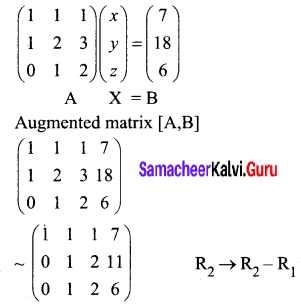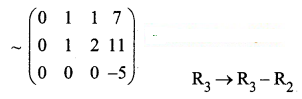The last equivalent matrix is in the echelon form. [A, B] has 3 non-zero rows and [A] has 2 non- zero rows.
ρ([A, B]) = 3, ρ(A) = 2
ρ(A) ≠ ρ([A, B])
Hence the given system is inconsistent and has no solution.

Question 5.
Find k if the equations 2x + 3y – z = 5, 3x – y + 4z = 2, x + 7y – 6z = k are consistent.
Solution:
The given system is 2x + 3y – z = 5, 3x – y + 4z = 2, x + 7y – 6z = k
It is also given that the system is consistent.
The matrix equation corresponding to the given system is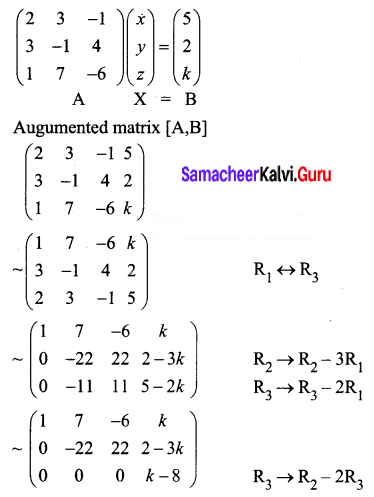ρ(A) = 2; ρ([A, B]) = 2 or 3
For the equations to be consistent, ρ([A, B]) = ρ(A) = 2
k – 8 = 0 ⇒ k = 8

Question 6.
Find k if the equations x + y + z = 1, 3x – y – z = 4, x + 5y + 5z = k are inconsistent.
Solution:
The given system is x + y + z = 1, 3x – y – z = 4, x + 5y + 5z = k and it is inconsistent.
The matrix equation corresponding to the given system is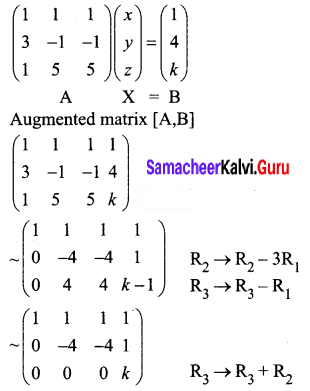ρ(A) = 2, since the equivalent matrix has 2 non zero rows.
For the equations to be inconsistent
ρ([A, B]) ≠ ρ(A)
ρ([A, B]) ≠ 2 ⇒ k ≠ 0
So k can assume any real value other than 0.

Question 7.
Solve the equations x + 2y + z = 7, 2x – y + 2z = 4, x + y – 2z = -1 by using Cramer’s rule.
Solution:
The given system is x + 2y + z = 7, 2x – y + 2z = 4, x + y – 2z = -1
Here ∆ = $$\left|\begin{array}{ccc} 1 & 2 & 1 \\ 2 & -1 & 2 \\ 1 & 1 & -2 \end{array}\right|$$
= 1(2 – 2) -2(4 – 2) + 1(2 + 1)
= 12 + 3
= 15 ≠ 0
We can apply Cramer’s ruleand the system is consistent and it has unique solution.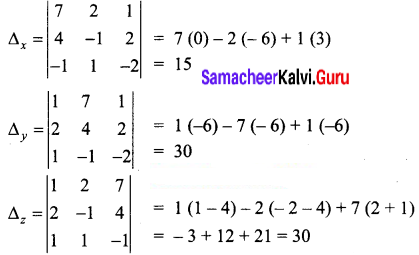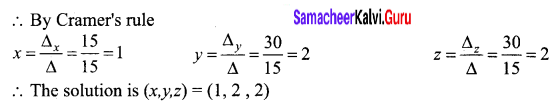Question 8.
The cost of 2kg. of wheat and 1kg. of sugar is ₹ 100. The cost of 1kg. of wheat and 1kg. of rice is ₹ 80. The cost of 3kg. of wheat, 2kg. of sugar and 1kg of rice is ₹ 220. Find the cost of each per kg., using Cramer’s rule.
Solution:
Let the cost of wheat per kg be ₹ x, cost of sugar per kg be ₹ y and cost of rice per kg be ₹ z, respectively.
It is given that 2x + y = 100, x + z = 80, 3x + 2y + z = 220
Here ∆ = $$\left|\begin{array}{lll} 2 & 1 & 0 \\ 1 & 0 & 1 \\ 3 & 2 & 1 \end{array}\right|$$
= 2 (-2) – 1(-2 )
= -2 ≠ 0
we can apply Cramer’s rule and the system is consistent and its has unique solution.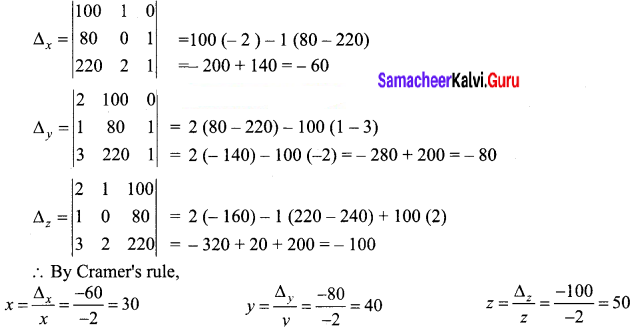Hence the cost of wheat is ₹ 30/kg, cost of sugar is ₹ 40/kg and the cost of rice is ₹ 50/kg.

Question 9.
A salesman has the following record of sales for three months for three items A,B and C, which have different rates of commission.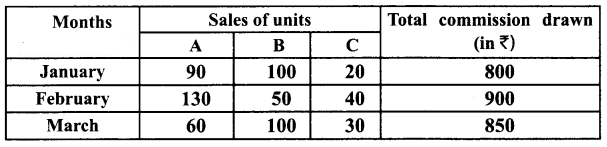Find out the rate of commission on the items A,B and C by using Cramer’s rule.
Solution:
Let the rate of commission on items A, B and C be ₹ x, ₹ y and ₹ z per unit respectively.
According to the problem we have,
January, 90x + 100y + 20z = 800
February, 130x + 50y + 40z = 900
March, 60x + 100y + 30z = 850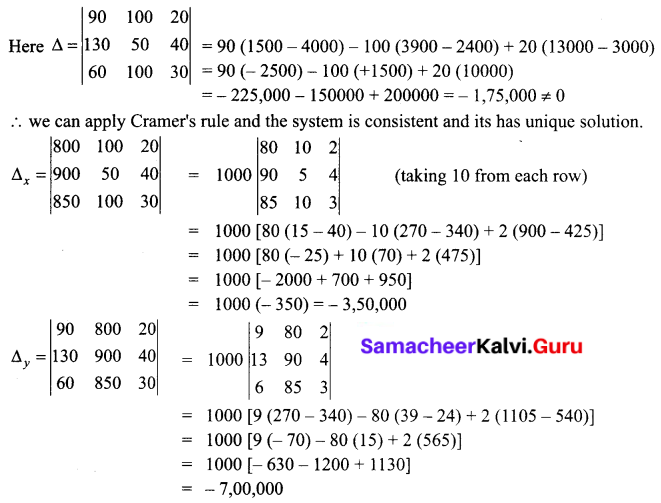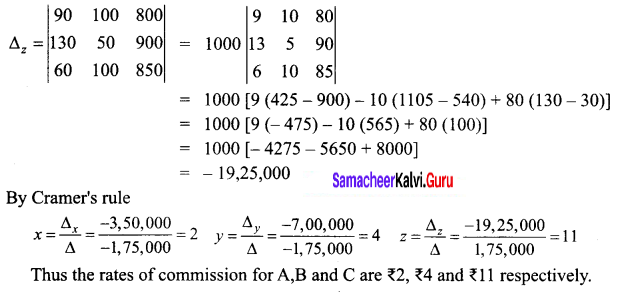Question 10.
The subscription department of a magazine sends out a letter to a large mailing list inviting subscriptions for the magazine. Some of the people receiving this letter already subscribe to the magazine while others do not. From this mailing list, 60% of those who already subscribe will subscribe again while 25% of those who do not now subscribe will subscribe. On the last letter it was found that 40% of those receiving it ordered a subscription. What percent of those receiving the current letter can be expected to order a subscription?
Solution:
Let X represent people who subscribe for the magazine and Y represent people who do not subscribe for the magazine. Then
(X X) ⇒ those who already subscribed will do it again.
(X Y) ⇒ those who already subscribed will not do it again.
(Y X) ⇒ those who have not subscribed will do it now.
(Y Y) ⇒ those who have not subscribed already will not do it now also.
From the question,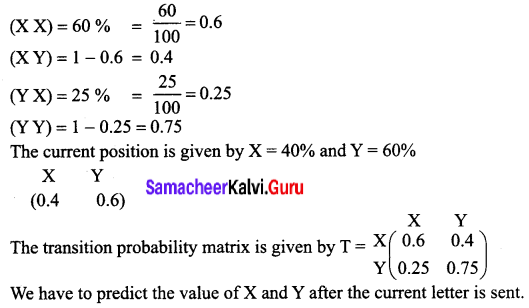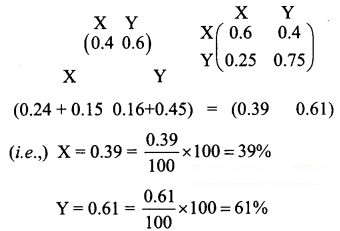Thus 39% of those receiving the current letter can be expected to order a subscription.